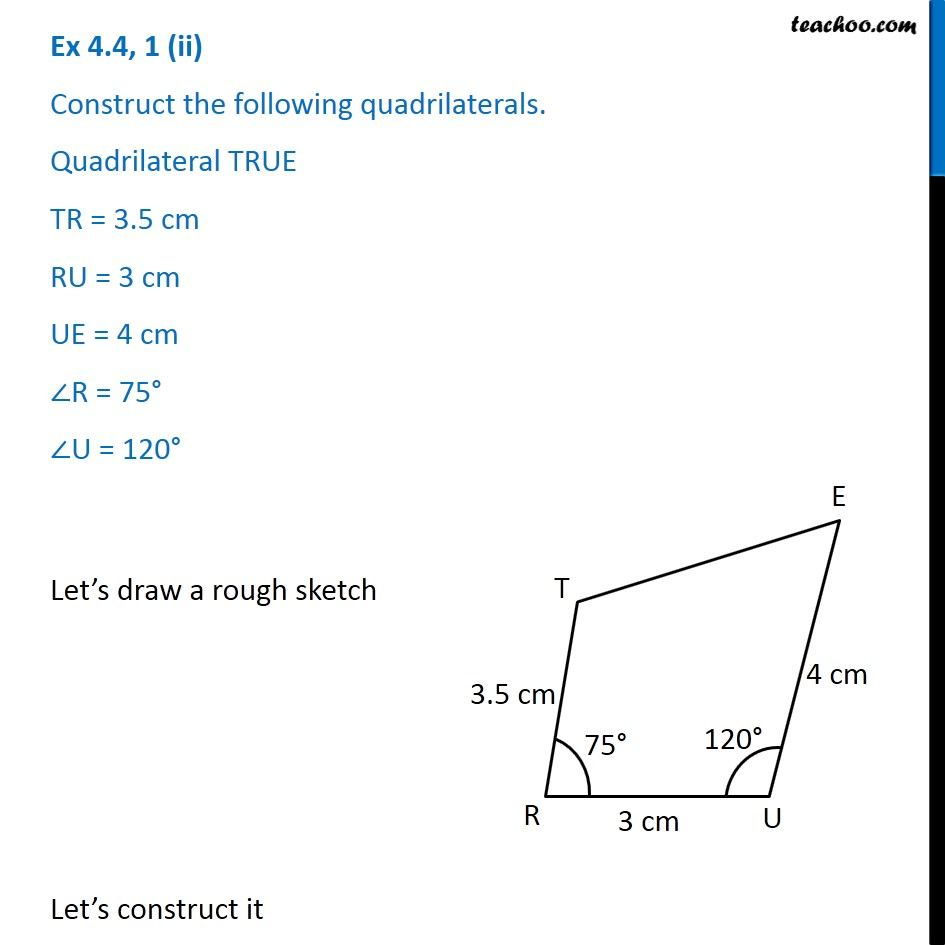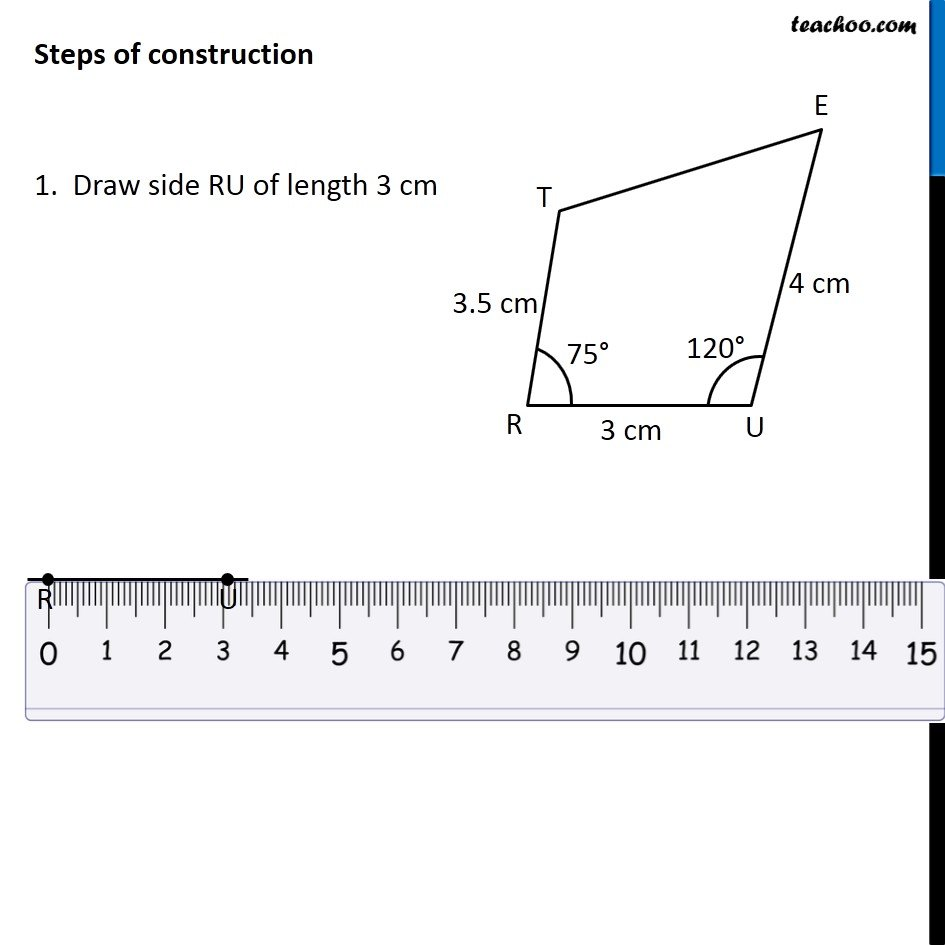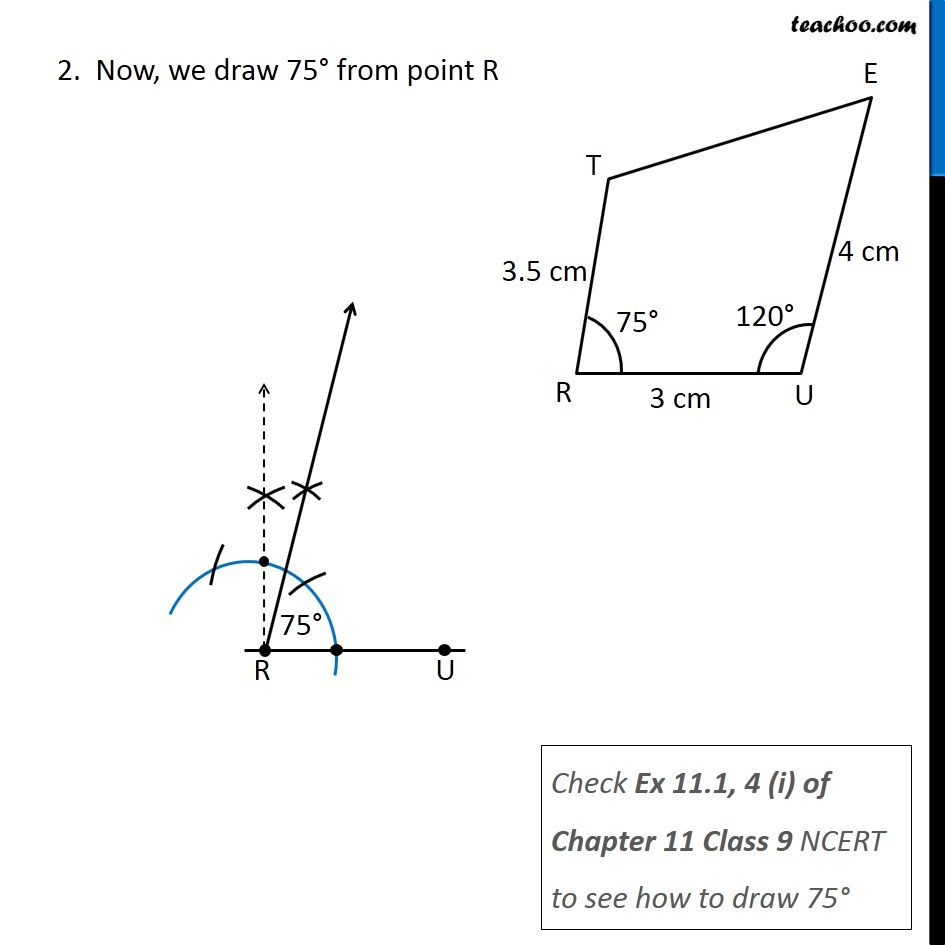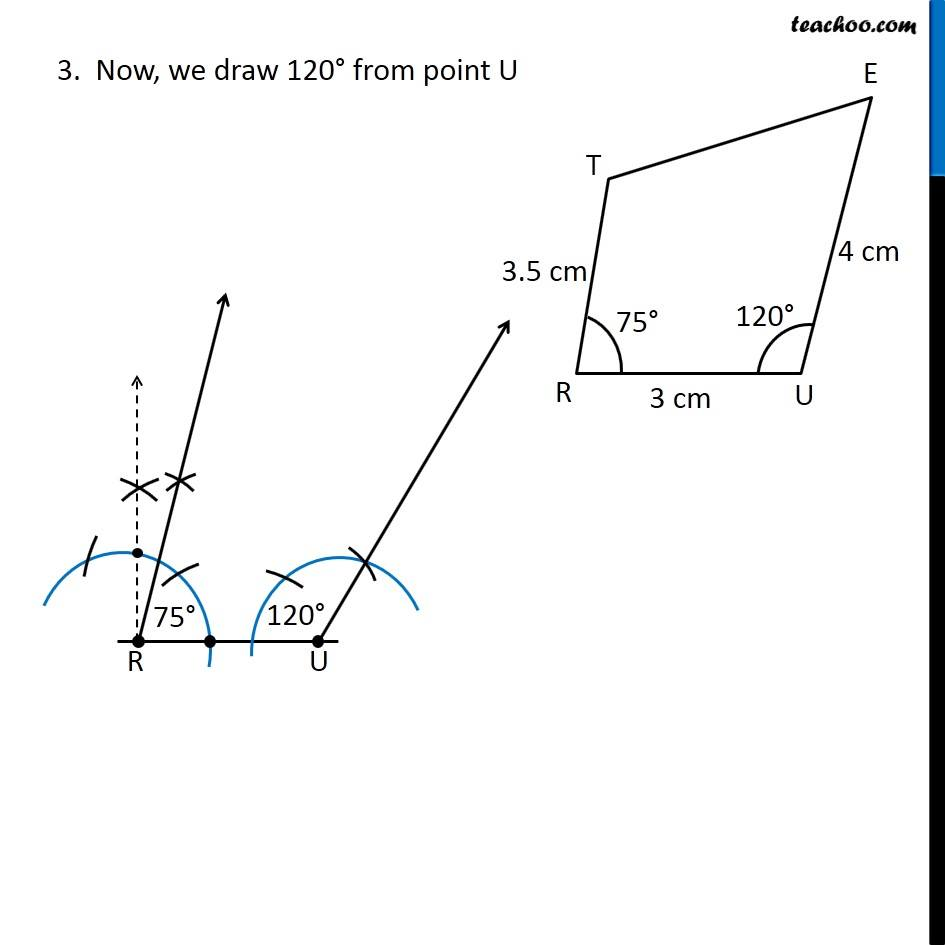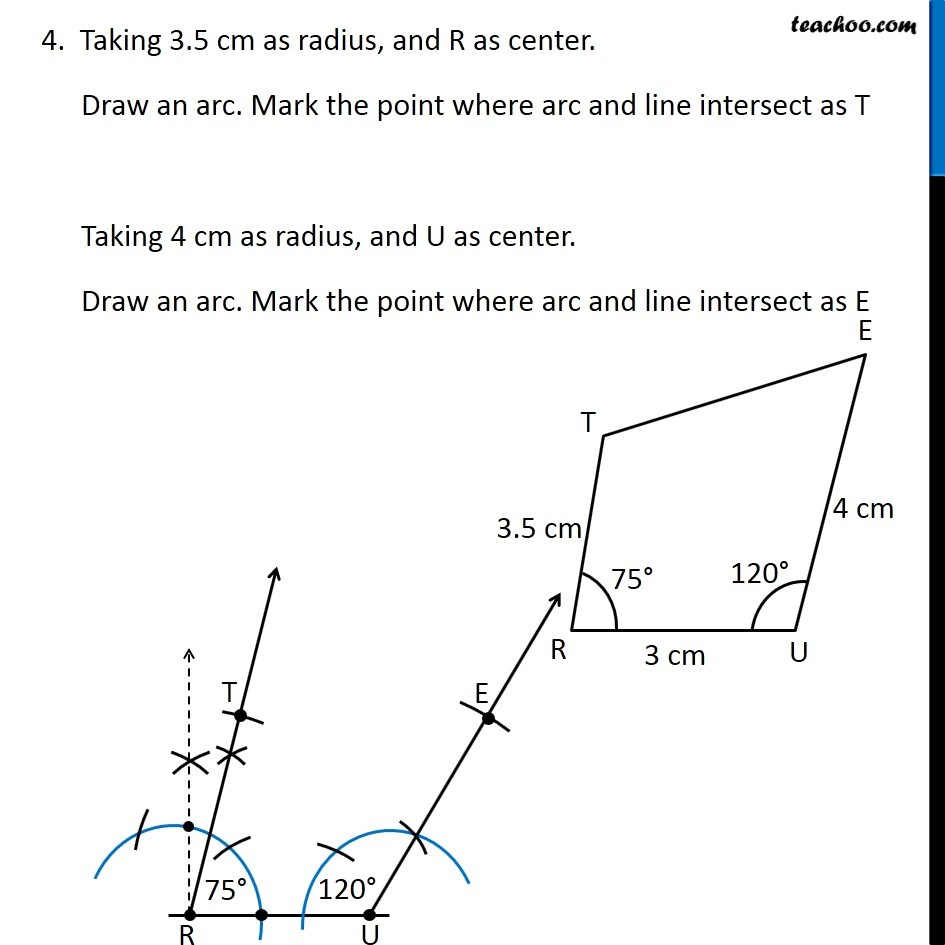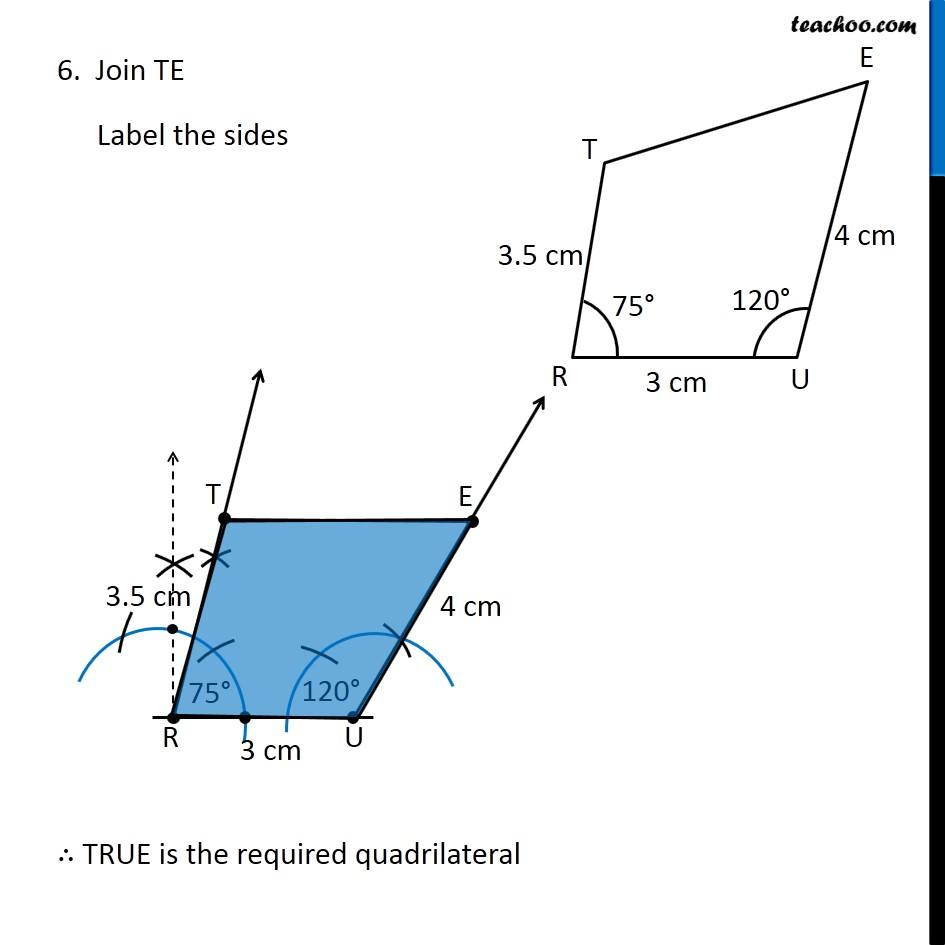1. Chapter 4 Class 8 Practical Geometry
2. Concept wise
3. Constructing Quadrilateral - 3 Sides and 2 angles given

Transcript

Ex 4.4, 1 (ii) Construct the following quadrilaterals. Quadrilateral TRUE TR = 3.5 cm RU = 3 cm UE = 4 cm ∠R = 75° ∠U = 120° Let’s draw a rough sketch Let’s construct it Steps of construction 1. Draw side RU of length 3 cm 2. Now, we draw 75° from point R Check Ex 11.1, 4 (i) of Chapter 11 Class 9 NCERT to see how to draw 75° 3. Now, we draw 120° from point U 4. Taking 3.5 cm as radius, and R as center. Draw an arc. Mark the point where arc and line intersect as T Taking 4 cm as radius, and U as center. Draw an arc. Mark the point where arc and line intersect as E 6. Join TE Label the sides

Constructing Quadrilateral - 3 Sides and 2 angles given